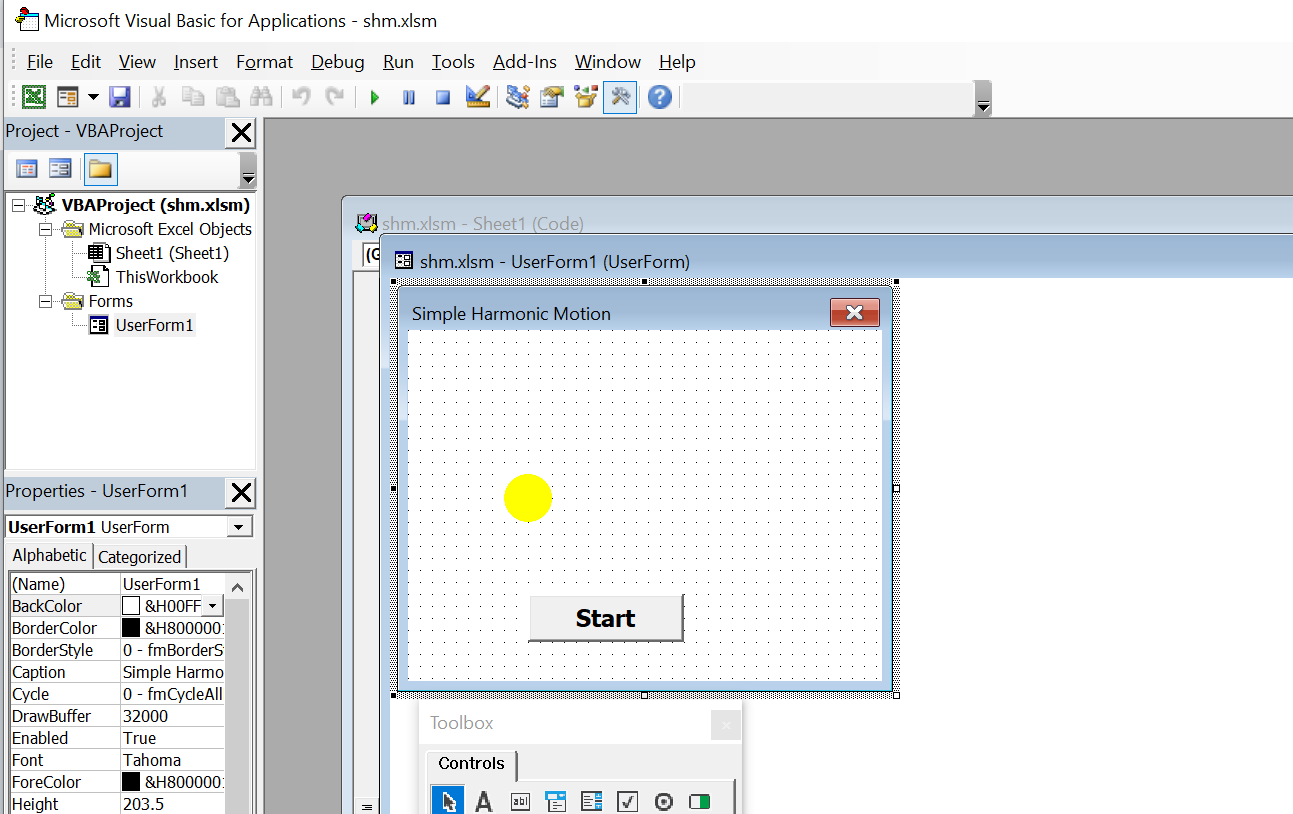# Simple Harmonic Motion

Simple harmonic motion is the motion of a simple harmonic oscillator. The motion is periodic, as it repeats itself at standard intervals in a specific manner with constant amplitude. It is characterized by its amplitude, its period(the time for a single oscillation), its frequency(the number of cycles per unit time), and its phase(which determines the starting point on the sine wave). The period, and its inverse the frequency, are constants determined by the overall system, while the amplitude and phase are determined by the initial conditions (position and velocity)of that system. (Wikipedia, 2008).

The equation of a simple harmonic motion is:

```x=Acos(2pft+f),
```

where x is the displacement, A is the amplitude of oscillation, f is the frequency, t is the elapsed time, and f is the phase of oscillation.

To create a simple model of simple harmonic motion in Excel VBA , use the equation x=Acos(wt), and assign a value of 500 to A and a value of 50 to w.The Design Interface In this example, insert a picture box and upload an image to the picture container. Next, insert a command button and change the captions to start. We can use the move method to move the image whose path is determined by the formula x = 500 * Cos(50 * t) . We use a Do Loop and the DoEvents command to create the motion.

When you run the program, the image will move in an oscillating motion as shown in the figure below:

#### The Code

```Private Sub Cmd_Start_Click()
Dim y As Integer
Dim t As Double
x = 0
Do

t = t + 1
y = 100 * Cos(50 * t)
Image1.Move Image1.Left + y

DoEvents

x = x + 2
Loop

End Sub
```